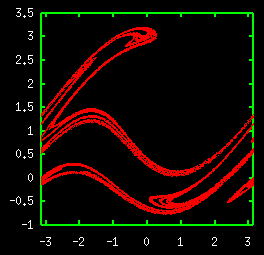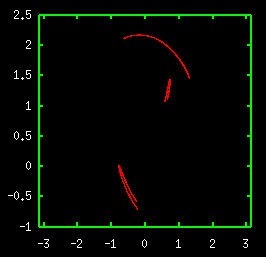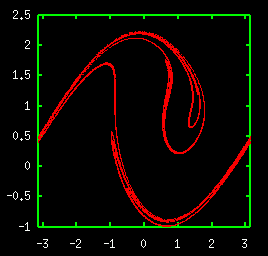# Attractors of Periodically Forced Pendulum

An attractor of periodically forced pendulum. The explanation is obtained in "periodically forced pendulum" animation.
The parameters are γ = 0.22 , ω =1.0, and a = 2.7.The next figure is a three band attractor of the same dynamical system.
The parameters are γ=1/√15, ω=0.65, and a=0.728.When the above three band attractor merges, one band attractor appears.
The parameters are γ=1/√15, ω=0.65, and a=0.75.
The bifurcation takes place at about a=0.728384.<< Attractor of Hénon Map / Bifurcation Diagram of Logistic Map >>

Introduction to Chaos and Nonlinear Dynamics